Notes for Level I CFA® Program

R54 Understanding Fixed-Income Risk and Return

3.4. Properties of Bond DurationWatch Video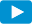The input variables for determining Macaulay and modified yield duration of fixed-rate bonds are:

• Coupon rate or payment per period
• Yield-to-maturity per period
• Number of periods to maturity
• Fraction of the period that has gone by

By changing one of the above variables while holding others constant, we can analyze the properties of bond duration, which in turn, helps us assess the interest rate risk. We will use the formula for Macaulay duration to understand the relationship between each variable and duration:The fraction of the coupon period that has gone by (t/T)
First, let us consider the relationship between fraction of time that has gone by (t/T) and duration. There is no change to the expression in braces {} as time passes by. Fraction of time (t/T) increases as time passes by. Assume T is 180. If 50 days have passed, then t/T = 0.277. If 90 days have passed, then t/T = 0.5. If 150 days have passed, then t/T = 0.83. As t/T increases from t = 0 to t = T with passing time, MacDuration decreases in value. Once the coupon is paid, t/T becomes zero and MacDuration jumps in value. When time to maturity is plotted against MacDuration, it creates a saw tooth pattern as shown graphically below:

Time-to-Maturity

The relationship between Macaulay Duration and time-to-maturity for four types of bonds (zero-coupon, discount, premium, and perpetuity) at t/T = 0 are shown.

• Zero-coupon bond: Pays no coupon so c = 0. Plugging in c = 0 and t/T = 0 in MacDur formula, we get Macaulay Duration = N. For a zero-coupon bond, duration is its time-to-maturity.
• Perpetuity: A perpetual bond is one that does not mature. There is no principal to redeem. It makes a fixed coupon payment forever. If N is a large number in the above equation, then the second part of the expression in the braces becomes zero. Denominator: N is an exponent.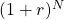is a very large number. So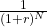must be zero and the value in the numerator will not matter here. Macaulay duration of a perpetual bond is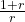as N approaches infinity.
• Premium bond: Bonds are trading at a premium above par or at par. The coupon rate is greater than or equal to yield-to-maturity (r). The numerator of the second expression in braces is always positive because c-r is positive. The denominator of the second expression in braces is always positive. Second expression as a whole is always positive. MacDuration = less thanbecause the second expression in braces is positive. As time passes, it approaches.
• Discount bond: For a discount bond, the coupon rate is below yield-to-maturity. The Macaulay Duration increases for a longer time-to-maturity. The numerator of the second expression in braces is negative because c-r is negative. Put together, duration at some point exceeds, reaches a maximum and approaches(the threshold) from above. This happens when N is large and coupon rate (c) is below the yield-to-maturity (r). As a result, for a long-term discount bond, interest rate risk can be lesser than a shorter-term bond.

The above points are summarized below:

 Relationship between bond duration and other input parameters Bond parameter Effect on Duration Higher coupon rate Lower Higher yield-to-maturity Lower Longer time-to-maturity Higher for a premium bond. Usually, holds true for a discount bond, but there can be exceptions. Exception: low coupon (relative to YTM) bond with long maturity.

Example 11: Calculating the approximate modified duration

A mutual fund specializes in investments in sovereign debt. The mutual fund plans to take a position on one of these available bonds.

 Bond Time-to-Maturity Coupon Rate Price Yield-to-Maturity (A) 5 years 10% 70.093879 20% (B) 10 years 10% 58.075279 20% (C) 15 years 10% 53.245274 20%

The coupon payments are annual. The yields-to-maturity are effective annual rates. The prices are per 100 of par value.

1. Compute the approximate modified duration of each of the three bonds using a 5 bps change in the yield-to-maturity and keeping precision to six decimals (because approximate duration statistics are very sensitive to rounding).
2. Which of the three bonds is expected to have the highest percentage price increase if the yield-to-maturity on each decreases by the same amount – for instance, by 10 bps from 20% to 19.90%?

Solution to 1:

Calculate PV+ and PV; then calculate modified duration.

Bond A:

PV0 = 70.093879;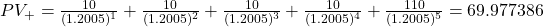PV+ = 69.977386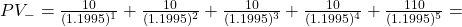70.210641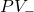= 70.210641

ApproxModDur =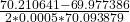= 3.33.

The approximate modified duration of Bond A is 3.33.

Bond B:

PV0 = 58.075279

PV+ =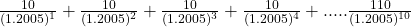= 57.937075
PV+ = 57.937075

PV=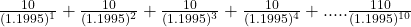= 58.213993
PV= 58.213993

ApproxModDur =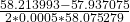= 4.77

The approximate modified duration of Bond B is 4.77.

Bond C:

PV0 = 53.245274

PV+ =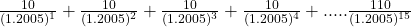= 53.108412
PV+ = 53.108412

PV =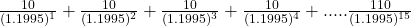= 53.382753
PV = 53.382753

ApproxModDur =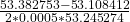= 5.15.

The approximate modified duration of Bond C is 5.15.

Solution to 2:

Bond C with 15 years-to-maturity has the highest modified duration. If the yield to maturity on each is decreased by the same amount – for instance, by 10bps, from 20% to 19.90% – Bond C would be expected to have the highest percentage price increase because it has the highest modified duration.

Interest Rate Risk Characteristics of Callable Bond

A callable bond is one that might be called by the issuer before maturity. This makes the cash flows uncertain. So, the YTM cannot be determined with certainty. The exhibit below plots the price-yield curve for a non-callable/straight bond and a callable bond. It also plots the change in price for a change in the benchmark yield curve. Bond price is plotted on the y-axis and the benchmark yield on the horizontal axis.

Interest Rate Risk Characteristics of Putable Bond